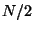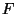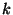## Multi-Dimensional Inverse Transform of Real Data

These are the hardest transforms to do because the symmetryhas to be put into the input array by hand for the modes where the frequency component in the last dimension isor the Nyquist frequency. If the data you are using were generated from a forward transform then these symmetries should already be in place and you don't have to worry about them, but if you want to generate your own multi-dimensional data in frequency space and then inverse transform them to a real function you have to be careful. This code initializes a three-dimensional array of complex numbers satisfying the necessary symmetries. Since this is a fairly common problem I invite you to simply copy this code verbatim and alter the lines where the values are set. There are eight such lines, all marked with the comment **Customize Here**. I also marked the line where the lattice spacingis set since this is a physical parameter that has to be set for each problem (as are the sizes,, andof course).

This particular code initializes the functionto(20)

The choice of function was basically arbitrary, but was guided by the following considerations. It falls off rapidly at large. This is good because the transform will have no information about large frequencies so if they are important the resultant data will probably be meaningless, or at least skewed. Also, this function does explicitly satisfy the symmetry condition, so if I were to take the inverse Fourier transform of this continuous function I would indeed get a real result.

This code doesn't produce any output because I couldn't think of anything useful to illustrate with the output. The point of the code was to illustrate how to set up the data with the correct symmetries.

#define N1 8 // Number of real points in the data - first dimension
#define N2 4 // Second dimension
#define N3 4 // Third dimension
void main()
{
int a,b,c;
int aconj,bconj; // Positions of the frequencies -a and -b on the lattice
float f[N1][N2][N3]; // An array of real numbers
float fnyquist[N1][2*N2];
int size[]={N1,N2,N3};
float ka,kb,kc,k2; // Frequency in each dimension and square magnitude of frequency
// The spacing dx is given as a parameter of the problem
// The spacing dk then gives the space between adjacent frequencies on the lattice
float dxa=3.5,dxb=2.3,dxc=1.4; **Customize Here**
float dka=1./dxa/(float)N1,dkb=1./dxb/(float)N2,dkc=1./dxc/(float)N3;

for(a=0;a<N1;a++) // Loop over first dimension
{
aconj=(a==0 ? 0 : N1-a); // Position on the lattice of frequency -a
ka=(a<=N1/2 ? a : a-N1); // Value of frequency in first dimension
ka *= dka; // Convert from index value to actual frequency
for(b=0;b<N2;b++) // Loop over second dimension
{
kb=(b<=N2/2 ? b : b-N2); // Value of frequency in second dimension
kb *= dkb;
// Set all modes with 0<c<N3/2. The complex conjugates of these modes do not need to be set.
for(c=1;c<N3/2;c++) // Main loop over last dimension
{
kc=c; // Value of frequency in last dimension
kc *= dkc;
k2 = ka*ka + kb*kb + kc*kc; // Set square magnitude of frequency
f[a][b][2*c]=cos(ka)/exp(k2); // Re(F_a,b,c) = cos(kx)/e^(k^2) **Customize Here**
f[a][b][2*c+1]=sin(ka)/exp(k2); // Im(F_a,b,c) = sin(kx)/e^(k^2) **Customize Here**
}
// Set modes with c=0 or c=N3/2.
// The complex conjugates of these modes appear explicitly on the lattice
//   and must be set to satisfy F(-k)=F(k)*
if(b>N2/2 || (a>N1/2 && (b==0 || b==N2/2))) // Set each mode only once
{
bconj=(b==0 ? 0 : N2-b); // Position on the lattice of frequency -b
// c=0
k2 = ka*ka + kb*kb; // Square magnitude of frequency
f[a][b]=cos(ka)/exp(k2); // Re(F_a,b,0) = cos(kx)/e^(k^2) **Customize Here**
f[a][b]=sin(ka)/exp(k2); // Im(F_a,b,0) = sin(kx)/e^(k^2) **Customize Here**
f[aconj][bconj]=f[a][b]; // Set complex conjugate mode
f[aconj][bconj]=-f[a][b];
// c=N3/2
kc = N3/2.*dkc;
k2 = ka*ka + kb*kb + kc*kc; // Square magnitude of frequency
fnyquist[a][2*b]=cos(ka)/exp(k2); // Re(F_a,b,N3/2) = cos(kx)/e^(k^2) **Customize Here**
fnyquist[a][2*b+1]=sin(ka)/exp(k2); // Im(F_a,b,N3/2) = sin(kx)/e^(k^2) **Customize Here**
fnyquist[aconj][2*bconj]=fnyquist[a][2*b]; // Set complex conjugate mode
fnyquist[aconj][2*bconj+1]=-fnyquist[a][2*b+1];
}
// The 8 "corners" of the lattice are set to real values
else if((a==0 || a==N1/2) && (b==0 || b==N2/2))
{
k2 = ka*ka + kb*kb; // Square magnitude of frequency for c=0
f[a][b]=cos(ka)/exp(k2); // Re(F_a,b,0) = cos(kx)/e^(k^2) **Customize Here**
f[a][b]=0.; // Imaginary part is set to 0
kc = N3/2.*dkc;
k2 = ka*ka + kb*kb + kc*kc; // Square magnitude of frequency for c=N3/2
fnyquist[a][2*b]=cos(ka)/exp(k2); // Re(F_a,b,N3/2) = cos(kx)/e^(k^2) **Customize Here**
fnyquist[a][2*b+1]=0.; // Imaginary part is set to 0
}
} // End of b loop (second dimension)
} // End of a loop (first dimension)

fftrn((float *)f,(float *)fnyquist,3,size,-1); // Inverse transform of 3-D data
}